Pandas Dataframe: Union and Concat Examples

# Pandas Dataframe: Union and Concat Examples

Last updated:

## SQL Union all

The default behaviour for pandas.concat is not to remove duplicates!

Use ignore_index=True to make sure sure the index gets reset in the new dataframe.

import pandas as pd

df1 = pd.DataFrame({
'name':['john','mary'],
'age':[24,45]
})

df2 = pd.DataFrame({
'name':['mary','john'],
'age':[45,89]
})

# pass dataframes as a list
pd.concat([df1,df2], ignore_index=True)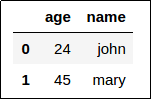Dataframe 1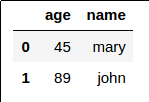Dataframe 2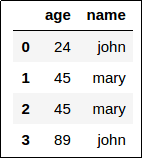Union of Dataframe 1 and 2:
Note that the index was reset and
the duplicate row was NOT removed

## SQL Union

In SQL, the union keyword implies that duplicates are removed:

import pandas as pd

df1 = pd.DataFrame({
'name':['john','mary'],
'age':[24,45]
})

df2 = pd.DataFrame({
'name':['mary','john'],
'age':[45,89]
})

pd.concat([
df1,df2
],ignore_index=True).drop_duplicates().reset_index(drop=True)Dataframe 1Dataframe 2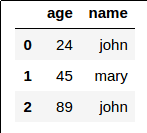Union of Dataframe 1 and 2:
No duplicates now

## Concatenate horizontally

To concatente dataframes horizontally (i.e. side-by-side) pd.concat() with axis=1:

import pandas as pd

df1 = pd.DataFrame({
'name':['john','mary'],
'age':[24,45]
})

df2 = pd.DataFrame({
'name':['mary','john'],
'age':[45,89]
})

pd.concat([
df1,df2
],axis=1)Dataframe 1Dataframe 2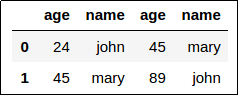Concatenation of Dataframe 1 and 2:
Pandas will not warn you if you try
to concatenate two dataframes that have
columns with the same name!

## Concatenate vertically

This is the same as applying SQL Union All